# PromQL Deep Dive - The Prometheus Query Language

Oct. 16, 2017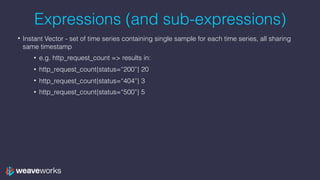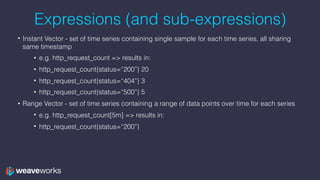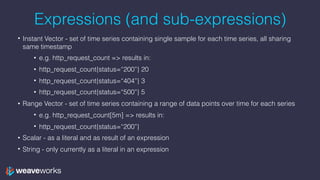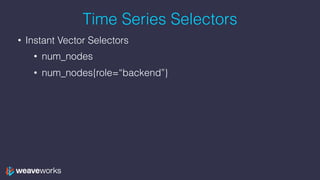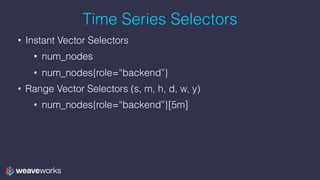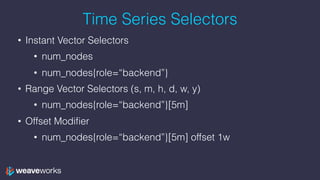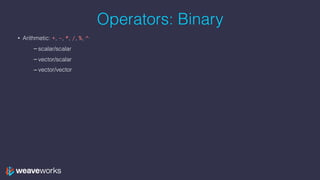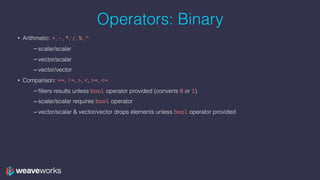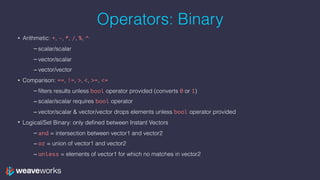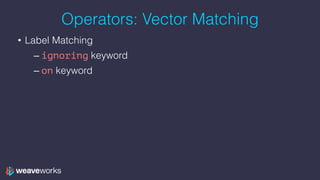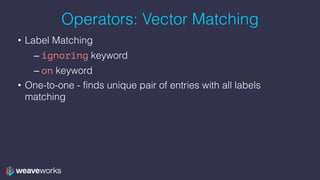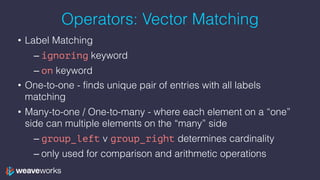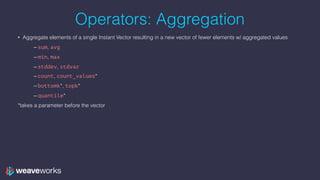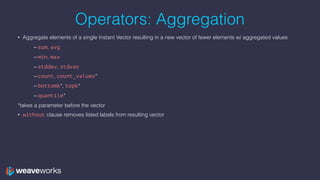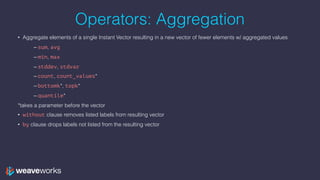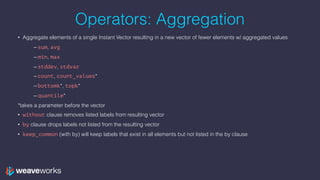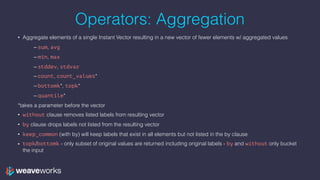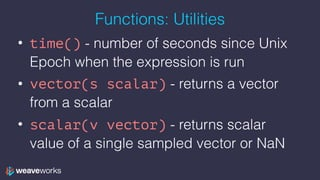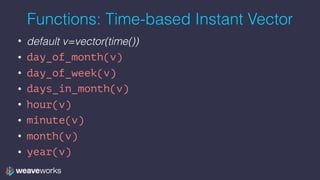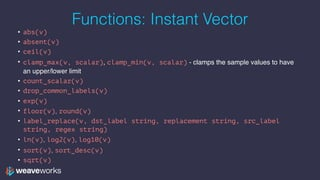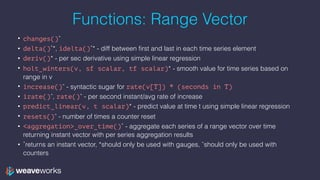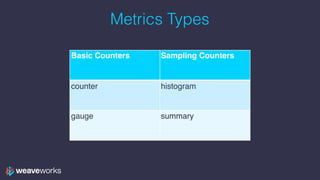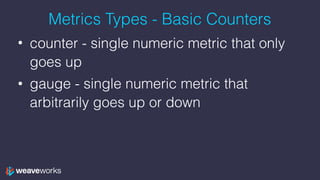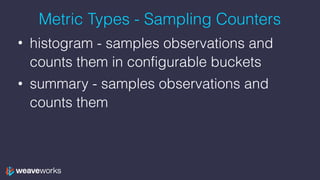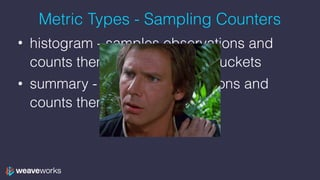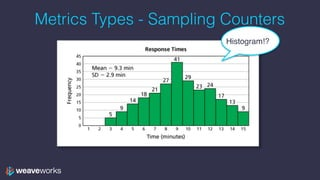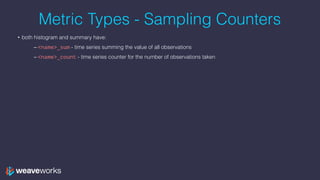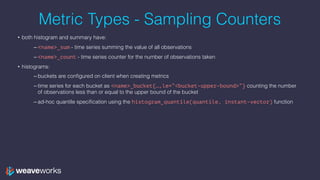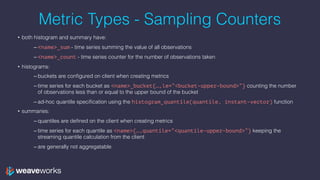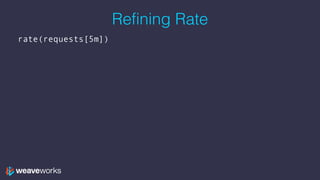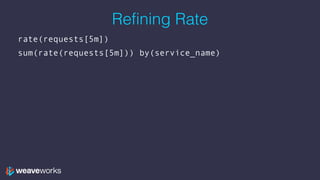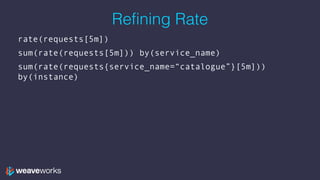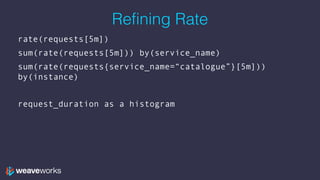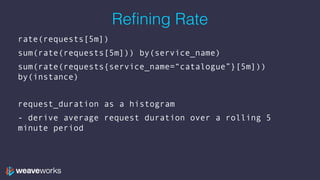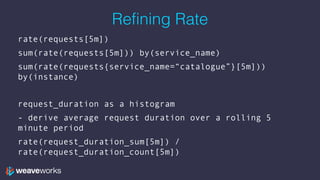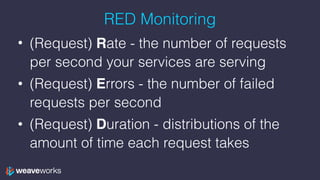1 of 43

### PromQL Deep Dive - The Prometheus Query Language

• 1. Prometheus: PromQL Deep Dive Jeff Hoffer, Developer Experience github.com/eudaimos
• 2. Agenda 1. What is PromQL 2. PromQL Operators 3. PromQL Functions 4. Hands On: Building Queries in PromQL 5. Hands On: Visualizing PromQL in Grafana 6. Training on real app 7. What’s next?
• 3. What is PromQL • Powerful Query Language of Prometheus • Provides built in operators and functions • Vector-based calculations like Excel • Expressions over time-series vectors
• 4. Expressions (and sub-expressions) • Instant Vector - set of time series containing single sample for each time series, all sharing same timestamp • e.g. http_request_count => results in: • http_request_count{status=“200”} 20 • http_request_count{status=“404”} 3 • http_request_count{status=“500”} 5
• 5. Expressions (and sub-expressions) • Instant Vector - set of time series containing single sample for each time series, all sharing same timestamp • e.g. http_request_count => results in: • http_request_count{status=“200”} 20 • http_request_count{status=“404”} 3 • http_request_count{status=“500”} 5 • Range Vector - set of time series containing a range of data points over time for each series • e.g. http_request_count[5m] => results in: • http_request_count{status=“200”}
• 6. Expressions (and sub-expressions) • Instant Vector - set of time series containing single sample for each time series, all sharing same timestamp • e.g. http_request_count => results in: • http_request_count{status=“200”} 20 • http_request_count{status=“404”} 3 • http_request_count{status=“500”} 5 • Range Vector - set of time series containing a range of data points over time for each series • e.g. http_request_count[5m] => results in: • http_request_count{status=“200”} • Scalar - as a literal and as result of an expression • String - only currently as a literal in an expression
• 7. Time Series Selectors • Instant Vector Selectors • num_nodes • num_nodes{role=“backend”}
• 8. Time Series Selectors • Instant Vector Selectors • num_nodes • num_nodes{role=“backend”} • Range Vector Selectors (s, m, h, d, w, y) • num_nodes{role=“backend”}[5m]
• 9. Time Series Selectors • Instant Vector Selectors • num_nodes • num_nodes{role=“backend”} • Range Vector Selectors (s, m, h, d, w, y) • num_nodes{role=“backend”}[5m] • Offset Modifier • num_nodes{role=“backend”}[5m] offset 1w
• 10. Operators: Binary • Arithmetic: +, -, *, /, %, ^ – scalar/scalar – vector/scalar – vector/vector
• 11. Operators: Binary • Arithmetic: +, -, *, /, %, ^ – scalar/scalar – vector/scalar – vector/vector • Comparison: ==, !=, >, <, >=, <= – filters results unless bool operator provided (converts 0 or 1) – scalar/scalar requires bool operator – vector/scalar & vector/vector drops elements unless bool operator provided
• 12. Operators: Binary • Arithmetic: +, -, *, /, %, ^ – scalar/scalar – vector/scalar – vector/vector • Comparison: ==, !=, >, <, >=, <= – filters results unless bool operator provided (converts 0 or 1) – scalar/scalar requires bool operator – vector/scalar & vector/vector drops elements unless bool operator provided • Logical/Set Binary: only defined between Instant Vectors – and = intersection between vector1 and vector2 – or = union of vector1 and vector2 – unless = elements of vector1 for which no matches in vector2
• 13. Operators: Vector Matching • Label Matching – ignoring keyword – on keyword
• 14. Operators: Vector Matching • Label Matching – ignoring keyword – on keyword • One-to-one - finds unique pair of entries with all labels matching
• 15. Operators: Vector Matching • Label Matching – ignoring keyword – on keyword • One-to-one - finds unique pair of entries with all labels matching • Many-to-one / One-to-many - where each element on a “one” side can multiple elements on the “many” side – group_left v group_right determines cardinality – only used for comparison and arithmetic operations
• 16. Operators: Aggregation • Aggregate elements of a single Instant Vector resulting in a new vector of fewer elements w/ aggregated values – sum, avg – min, max – stddev, stdvar – count, count_values* – bottomk*, topk* – quantile* *takes a parameter before the vector
• 17. Operators: Aggregation • Aggregate elements of a single Instant Vector resulting in a new vector of fewer elements w/ aggregated values – sum, avg – min, max – stddev, stdvar – count, count_values* – bottomk*, topk* – quantile* *takes a parameter before the vector • without clause removes listed labels from resulting vector
• 18. Operators: Aggregation • Aggregate elements of a single Instant Vector resulting in a new vector of fewer elements w/ aggregated values – sum, avg – min, max – stddev, stdvar – count, count_values* – bottomk*, topk* – quantile* *takes a parameter before the vector • without clause removes listed labels from resulting vector • by clause drops labels not listed from the resulting vector
• 19. Operators: Aggregation • Aggregate elements of a single Instant Vector resulting in a new vector of fewer elements w/ aggregated values – sum, avg – min, max – stddev, stdvar – count, count_values* – bottomk*, topk* – quantile* *takes a parameter before the vector • without clause removes listed labels from resulting vector • by clause drops labels not listed from the resulting vector • keep_common (with by) will keep labels that exist in all elements but not listed in the by clause
• 20. Operators: Aggregation • Aggregate elements of a single Instant Vector resulting in a new vector of fewer elements w/ aggregated values – sum, avg – min, max – stddev, stdvar – count, count_values* – bottomk*, topk* – quantile* *takes a parameter before the vector • without clause removes listed labels from resulting vector • by clause drops labels not listed from the resulting vector • keep_common (with by) will keep labels that exist in all elements but not listed in the by clause • topk/bottomk - only subset of original values are returned including original labels - by and without only bucket the input
• 21. Functions: Utilities • time() - number of seconds since Unix Epoch when the expression is run • vector(s scalar) - returns a vector from a scalar • scalar(v vector) - returns scalar value of a single sampled vector or NaN
• 22. Functions: Time-based Instant Vector • default v=vector(time()) • day_of_month(v) • day_of_week(v) • days_in_month(v) • hour(v) • minute(v) • month(v) • year(v)
• 23. Functions: Instant Vector • abs(v) • absent(v) • ceil(v) • clamp_max(v, scalar), clamp_min(v, scalar) - clamps the sample values to have an upper/lower limit • count_scalar(v) • drop_common_labels(v) • exp(v) • floor(v), round(v) • label_replace(v, dst_label string, replacement string, src_label string, regex string) • ln(v), log2(v), log10(v) • sort(v), sort_desc(v) • sqrt(v)
• 24. Functions: Range Vector • changes()ˆ • delta()˚*, idelta()˚* - diff between first and last in each time series element • deriv()* - per sec derivative using simple linear regression • holt_winters(v, sf scalar, tf scalar)* - smooth value for time series based on range in v • increase()ˆ - syntactic sugar for rate(v[T]) * (seconds in T) • irate()ˆ, rate()ˆ - per second instant/avg rate of increase • predict_linear(v, t scalar)* - predict value at time t using simple linear regression • resets()ˆ - number of times a counter reset • <aggregation>_over_time()˚ - aggregate each series of a range vector over time returning instant vector with per series aggregation results • ˚returns an instant vector, *should only be used with gauges, ˆshould only be used with counters
• 25. Metrics Types Basic Counters Sampling Counters counter histogram gauge summary
• 26. Metrics Types - Basic Counters • counter - single numeric metric that only goes up • gauge - single numeric metric that arbitrarily goes up or down
• 27. Metric Types - Sampling Counters • histogram - samples observations and counts them in configurable buckets • summary - samples observations and counts them
• 28. Metric Types - Sampling Counters • histogram - samples observations and counts them in configurable buckets • summary - samples observations and counts them
• 29. Metrics Types - Sampling Counters Histogram!?
• 30. Metric Types - Sampling Counters • both histogram and summary have: – <name>_sum - time series summing the value of all observations – <name>_count - time series counter for the number of observations taken
• 31. Metric Types - Sampling Counters • both histogram and summary have: – <name>_sum - time series summing the value of all observations – <name>_count - time series counter for the number of observations taken • histograms: – buckets are configured on client when creating metrics – time series for each bucket as <name>_bucket{…,le=“<bucket-upper-bound>”} counting the number of observations less than or equal to the upper bound of the bucket – ad-hoc quantile specification using the histogram_quantile(quantile, instant-vector) function
• 32. Metric Types - Sampling Counters • both histogram and summary have: – <name>_sum - time series summing the value of all observations – <name>_count - time series counter for the number of observations taken • histograms: – buckets are configured on client when creating metrics – time series for each bucket as <name>_bucket{…,le=“<bucket-upper-bound>”} counting the number of observations less than or equal to the upper bound of the bucket – ad-hoc quantile specification using the histogram_quantile(quantile, instant-vector) function • summaries: – quantiles are defined on the client when creating metrics – time series for each quantile as <name>{…,quantile=“<quantile-upper-bound>”} keeping the streaming quantile calculation from the client – are generally not aggregatable
• 33. Refining Rate rate(requests[5m])
• 34. Refining Rate rate(requests[5m]) sum(rate(requests[5m])) by(service_name)
• 35. Refining Rate rate(requests[5m]) sum(rate(requests[5m])) by(service_name) sum(rate(requests{service_name=“catalogue”}[5m])) by(instance)
• 36. Refining Rate rate(requests[5m]) sum(rate(requests[5m])) by(service_name) sum(rate(requests{service_name=“catalogue”}[5m])) by(instance) request_duration as a histogram
• 37. Refining Rate rate(requests[5m]) sum(rate(requests[5m])) by(service_name) sum(rate(requests{service_name=“catalogue”}[5m])) by(instance) request_duration as a histogram - derive average request duration over a rolling 5 minute period
• 38. Refining Rate rate(requests[5m]) sum(rate(requests[5m])) by(service_name) sum(rate(requests{service_name=“catalogue”}[5m])) by(instance) request_duration as a histogram - derive average request duration over a rolling 5 minute period rate(request_duration_sum[5m]) / rate(request_duration_count[5m])
• 39. RED Monitoring • (Request) Rate - the number of requests per second your services are serving • (Request) Errors - the number of failed requests per second • (Request) Duration - distributions of the amount of time each request takes
• 40. Training!
• 41. Join the Weave user group! meetup.com/pro/Weave/  weave.works/help
• 42. Other topics • Kubernetes 101 • Continuous delivery: hooking up my CI/CD pipeline to Kubernetes • Network policy for security We have talks on all these topics in the Weave user group!
• 43. Thanks! Questions? We are hiring! DX in San Francisco Engineers in London & SF weave.works/weave-company/hiring